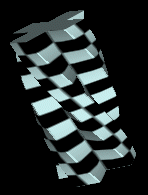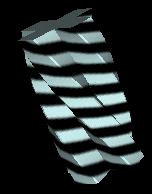# Texture Routines

In addition to the default TexGen modes that are supplied by OpenGL, the tubing library also contains some of its own automatic texture coordinate generation routines. This is needed in order to be able to draw a texture that 'sticks' to the extruded surface. The GLE library supplies 12 different texture mapping modes; in addition, user-defined texture coord generation routines can be supplied. (See the pipeline documentation for details). GLE needs to specify these many routines, since no one method is appropriate for all shapes. The 'flat' texture modes are appropriate for flat, open surfaces. The 'cylinder' texture modes are appropriate for long spaghetti shapes. The 'sphere' modes are appropriate for ball-like surfaces of revolution. Use the 'vertex' versions of these routines if your surface is nearly isometric (i.e. when the distances between the points in the contour are roughly equal to the distances between these same points in final 3D shape.) Use the 'normal' versions of these routines when changes in the normal vector are a better indicator of the distance between points on the surface. (In the case of a cylinder, one should expect that 'vertex' and 'normal' texturing yield the same results.) The 'model' modes will in general pin the texture onto the surface, and so the surface wiggles, it will look like the texture is painted on. The non-model modes will use real-space coordinates, and so the texture may fit better on static, fixed shapes; however, if the shape wiggles, then the texture will squirm around on the surface. You'll need to pick an appropriate mode for your shape. If you're clever, then define your own. (and send me patches if possible).

To use GLE texture mapping with the extrusion library, one must remember to "do the obvious":

• Enable texture mapping through OpenGL
• Define and load (lmdef/lmbind) a texture
• If you enable gleTextures, then disable glTexgGen
`gleTextureMode(int mode);   /* bitwise OR of flags */`
This routine can be used to set the type of automatic texture coordinate generation to be used. The argument should be a bitwise-OR of any of the following flags:
GLE_TEXTURE_ENABLE
If this bit is set, then texturing is enabled. If this bit is NOT set, then automatic texture coordinate generation is disabled.

The way in which the automatic texture coordinate generation occurs is determined by one of the following flags. One and only one of these should be selected at a time. These tokens are enumerants, not bit-flags.
GLE_TEXTURE_VERTEX_FLAT
Uses the vertexes "x" coordinate as the texture "u" coordinate, and the accumulated segment length as the "v" coordinate.

GLE_TEXTURE_NORMAL_FLAT
Uses the normal vector's "x" coordinate as the texture "u" coordinate, and the accumulated segment length as the "v" coordinate.

GLE_TEXTURE_VERTEX_CYL
Uses u = phi/(2*pi) = arctan (vy/vx)/(2*pi) as the texture "u" coordinate, and the accumulated segment length as the "v" coordinate. In the above equation, "vx" and "vy" stand for the vertex's x and y coordinates.

GLE_TEXTURE_NORMAL_CYL
Uses u = phi/(2*pi) = arctan (ny/nx)/(2*pi) as the texture "u" coordinate, and the accumulated segment length as the "v" coordinate. In the above equation, "nx" and "ny" stand for the normal's x and y coordinates.

GLE_TEXTURE_VERTEX_SPH
Uses u = phi/(2*pi) = arctan (vy/vx)/(2*pi) as the texture "u" coordinate, and v = theta/pi = (1.0 - arccos(vz))/pi as the texture "v" coordinate. In the above equation, "vx","vy" and "vz" stand for the vertex's x, y and z coordinates.

GLE_TEXTURE_NORMAL_SPH
Uses u = phi/(2*pi) = arctan (ny/nx)/(2*pi) as the texture "u" coordinate, and v = theta/pi = (1.0 - arccos(nz))/pi as the texture "v" coordinate. In the above equation, "nx","ny" and "nz" stand for the normal's x, y and z coordinates.

GLE_TEXTURE_VERTEX_MODEL_FLAT
GLE_TEXTURE_NORMAL_MODEL_FLAT
GLE_TEXTURE_VERTEX_MODEL_CYL
GLE_TEXTURE_NORMAL_MODEL_CYL
GLE_TEXTURE_VERTEX_MODEL_SPH
GLE_TEXTURE_NORMAL_MODEL_SPH
These define texture mapping modes that are very similar to those described above, except that the untransformed vertices and/or normals are used. As a result, textures tends to stick to the extrusion according to the extrusions local surface coordinates rather than according to real-space coordinates. This will in general provide the correct style of texture mapping when affine transforms are being applied to the contour, since the coordinates used are those prior to the affine transform.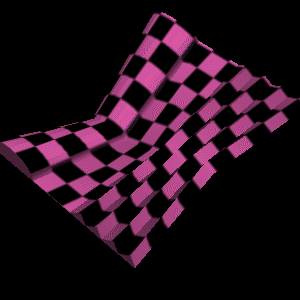## How it Works

To best understand how to use the above functions, it is best to understand how the tubing is actually drawn. Let us start by defining some terms. The tubing library "extrudes" a "contour" along a "path". The contour is a 2D polyline. The path is a 3D polyline. We use the word "segment" to refer to a straight-line segment of the path polyline. We also interchangeably use the word "segment" to stand for the section of the extrusion that lies along a path segment.

The tubing library draws segments one at a time. It uses glPushmatrix() and glPopmatrix() to orient each segment along the negative z-axis. The segment starts at z=0 and ends at some negative z-value (equal to the length of the segment). The segment is then drawn by calling glVertex3f() (and glNormal3F()) by drawing the 2D contour at z=0 and again at z=-len. (Of course, if the join style is one of the fancy ones, then the end-points are trimmed in a variety of ways, and do not land exactly on z=0, or z=-len, but they do come close). Note that glBegin() and glEnd() are called around each segment. (Note also that additional glBegins/Ends may be called to draw end-caps or filleting triangles for the more complex join styles.)

The obvious way to automatically generate textures is to warp the glVertex() and glNormal() functions, and compute texture coordinates based on the 3-space vertex and normal coordinates. This is essentially what the tubing code does, except that it passes some extra parameters. The glBegin calls are wrapped, and the integer segment number and the floating-point length of the segment are passed in. By knowing the segment number, and the segment length, the texture coordinates can be adjusted. Knowing the length allows the length to be accumulated, so that a texture is applied lengthwise along the extrusion. It is this accumulated length that is used in the FLAT and CYL mapping modes.

For each vertex, not only are the vertex x,y,z coordinates available, but so is a contour vertex counter indicating which contour vertex this corresponds to. There is also a flag indicating whether the vertex corresponds to a front or back vertex (i.e. a z=0 or z=-len vertex). Again, this info can be used to avoid confusion when drawing the more complex join styles.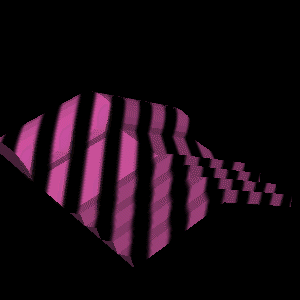## Hints, Tips and Techniques

• Hint: Confused? RUN THE DEMOS! The best way to understand what all the different texture modes are doing is to see them in action.
• Hint: The texture matrix can be used to your advantage! That is, you can use glMatrixMode(GL_TEXTURE) to control how textures are mapped to the surface. In particular, you may/will want to use it to to rescale the V coordinate.
• The origin of the contour will in general change the vertex x's and y's, thus changing the texture coordinates.
• The contour "up" vector will NOT influence the texture coordinates.
• For the FLAT and CYL modes, the accumulated length really is the accumulated length of the segments in modeling coordinates. Unless the extrusion is very small, this length will probably be much larger than 1.0, and so the resulting texture coordinate will wrap. You will generally want to rescale the "V" coordinate to make the texture map fit.
• If the texture is "swimming" around on the surface in an undesired way, try using the "MODEL" version of the texture generation flag.
• Typically, you will NOT want to use the "SPH" versions of the texture generation engine unless you really, really have an extrusion for which spherical coordinates are appropriate. Most uses of extrusions are best handled with the "FLAT" and "CYL" generation methods.
• User-defined texture generation callbacks are not currently implemented, but these should be very, very easy to hack in as desired. It should be easy to let your imagination run wild in here. Look at texgen.c -- what needs to be done should be obvious, I hope. When in doubt, experiment.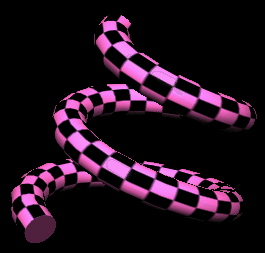The End
Version 2.2.1 -- clean up texture documentation.